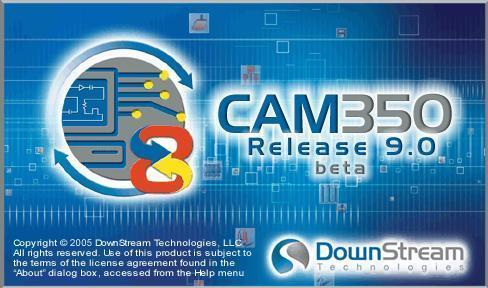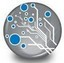# CAM350快捷键与命令有哪些-CAM350快捷键与命令大全

在使用CAM350的过程中，经常就会用到快捷键，那么大家知道CAM350快捷键与命令有哪些吗?下面准备就为大家带来了CAM350快捷键与命令大全，有需要的朋友可以来看看哦。字母键的应用

Q: 查看D码和刀具，并设为当前

W: 无选择命令时是框选放大窗口,有选择命令时为框选

R: 刷新

T: 透明显示

Y: 打开层列表

U: 撤消

I: 无选择命令时没作用,有选择命令时前面+W为反选

O: 有角度命令时为:04590度间相互切换

P: 返回上次视窗

A: 打开D码表

S: 光标随格点移动

D: 打开D码表并设为当前D码

F: 一维线二维线和零线宽间相互切换

G: 打开小窗口无作用

H: 高亮显示当前D码或刀具

K: 关闭某一层当前层除外

L: 打开某一层并设为当前层

Z: 打开自动捕捉中点

X: 光标切换

C: 无选择命令时是放大窗口并光标在视窗中央,有选择命令前面+W为框住部分就能选择整个物体或网络

V: 打开格点显示

N: 负片显示

“ ,”: 重复上次命令

Home: 全屏显示

Page UP: 放大捕捉中心

Page Down: 缩小捕捉中心

Insert 光标反主向移动窗口

文件

Alt+F+N 新建

Alt+F+O 打开

Alt+F+S 保存

Alt+F+M 合并菲林

Alt+F+I+U 自动导入

Alt+F+I+G 导入Gerber

Alt+F+I+R 导入钻带

Alt+F+I+M 导入锣带

Alt+F+E+G导出Gerber

Alt+F+E+C 导出复合层

Alt+F+E+R 导出钻带

Alt+F+E+M 导出锣带

Alt+F+P+P 打印

编辑

U 撤消(可连续撤消16步)

Ctrl+U 恢复(可连续操作16步)

Alt+E+M 移动

Alt+E+C 复制

Alt+E+D 删除

Alt+E+R 旋转

Alt+E+I 镜像

Alt+E+L+A 增加层

Alt+E+L+R 删除层

Alt+E+L+O 层排序

Alt+E+L+L 层对齐

Alt+E+L+T 以钻孔校正焊盘

Alt+E+L+S 层比例缩放(做字符高度不够时用)

Alt+E+H+D 更改D码

Alt+E+H+T+T 更改文字

Alt+E+H+E+A 打散所有

Alt+E+H+S 打散圆弧

Alt+E+H+O+S 改变原点

Alt+E+T+L 线剪切

Alt+E+T+C 圆剪切

Alt+E+N+J 连接节点

Alt+E+V 移动节点

Alt+E+A 增加节点Alt+E+E

删除节点

Alt+E+G 删除线段

增加

Alt+A+F 增加焊盘

Alt+A+L 增加线

Alt+A+P 增加多边形(做电镀边、阻流点和网格用)

Alt+A+X 增加文字

Alt+A+R 增加矩形

Alt+A+C+C 中心半径画圆

Alt+A+A+3 三点画弧

视图

Alt+V+C 查看复合层

信息

Alt+I+M+P 测量点到点的距离(中心到中心)

Alt+I+M+O测量物到物的距离(边沿到边沿，两物体间的最小距离)

Alt+I+R+D 查看D码信息(做文字宽度时用)

效用

Alt+U+R 线转自定义

Alt+U+D+I线转焊盘手动转

Alt+U+Y+D 提取大铜皮

Alt+U+Y+R 填充大铜皮

Alt+U+O+C 删除重复数据

Alt+U+O+I 删除独立焊盘(内层正片用)

Alt+U+T 添加泪滴

Alt+U+Z 整体缩放

Alt+U+C 快速生成复合层

分析

Alt+N+R DRC检测(查看线宽、线距是否符合本厂的工艺工求)

Alt+N+O 计算铜皮面积(电镀面积=铜皮面积/PANL的面积*100%)

表格

Alt+O+N 进入NC编辑

Alt+T+C编辑复合层

设置

Alt+S+U 设置单位

Alt+S+T 设置字符

Alt+M+M 更多宏命令 下面是进入NC编辑后的命令

Alt+E+L+N 新增NC层(注：增加时一定要选取对应的刀具表)

Alt+E+H+I+A 增加锣带交点(锣带变形时用)

Alt+E+H+F 改变钻孔的先后钻

Alt+E+H+H 改变钻孔

Alt+E+S 删除锣带线段

Alt+A+H 增加钻孔

Alt+A+S增加槽孔

Alt+A+C 增加扩孔

Alt+U+F 偏移锣带路径(也叫锣刀补偿)

Alt+U+O 优化钻孔

Alt+U+D Gerber转钻孔

Alt+N+D 检测钻孔

以上就是小编带来的CAM350快捷键与命令大全，希望能够帮助大家更加方便快捷地使用CAM350哦。